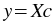## Creating Linear Fit Plugins

To create a linear fit plugin, you could implement your own fitting algorithms and output the appropriate vectors. However, Kst already comes with header files that make it easy for you to implement linear least-squares fit plugins by just providing a few functions. This section will describe how to take advantage of these files.

Two header files are provided for performing linear fits, `linear.h` (for unweighted linear fits) and `linear_weighted.h` (for weighted linear fits). They are both located under `kst/plugins/fits/` in the Kst source tarball. To use these files, include only one of them in the source code for your plugin:

```#include <../linear.h>
```

or

```#include <../linear_weighted.h>
```

(by convention, we will place the source code for the plugin one directory below where the header files are).

### Implementing Required Functions

Given a general linear model:where `y` is a vector of `n` observations, `X` is an `n` by `p` matrix of predictor variables, and `c` is the vector of `p` best-fit parameters that are to be estimated, the header files provide functions for estimating `c` for a given `y` and `X`. To provide `X`, the following function needs to be implemented in the source code for the plugin:

`double calculate_matrix_entry( double dX, int iPos )`

This function should return the value of the entry in column `iPos` of the matrix of predictor variables, using `x` value `dX`. This function will be called by linear.h or linear_weighted.h. The implementation of this function depends on the model you wish to use for the fit, and is unique to each linear fit plugin. For example, to fit to a polynomial model, `calculate_matrix_entry` could be implemented as follows:

```double calculate_matrix_entry( double dX, int iPos ) {
double dY;
dY = pow( dX, (double)iPos );
return dY;
}
```

### Calling the Fitting Functions

Once the appropriate header file has been included and `calculate_matrix_entry` has been implemented, call the appropriate fitting function included from the header file:

``````kstfit_linear_unweighted( inArrays, inArrayLens,
outArrays, outArrayLens,
outScalars, iNumParams )```;
```

or

``````kstfit_linear_weighted( inArrays, inArrayLens,
outArrays, outArrayLens,
outScalars, iNumParams )```;
```

Each function will return `0` on success, or `-1` on error, so it is a good idea to set the return value of the exported C function to be equal to the return value of the fitting function. To maintain simplicity, the code for the plugin can simply pass the arguments given to the exported C function to the fitting function. Note, however, that inArrays must be structured as follows:

• `inArrays` must contain the array of x coordinates of the data points

• `inArrays` must contain the array of y coordinates of the data points

• `inArrays` only exists if `kstfit_linear_weighted` is being called, and must contain the array of weights to use for the fit.

The easiest way to ensure that inArrays is structured correctly is to specify the correct order of input vectors in the XML file for the plugin.

`iNumParams` is the number of parameters in the fitting model used, which should be equal to the number of columns in the matrix `X` of predictor variables. `iNumParams` must be set correctly before the fitting function is called.

After `kstfit_linear_unweighted` or `kstfit_linear_weighted` is called, `outArrays` and `outScalars` will be set as follows:

• `outArrays` will contain the array of fitted y values.

• `outArrays` will contain the array of residuals.

• `outArrays` will contain the array of best-fit parameters that were estimated.

• `outArrays` will contain the covariance matrix, returned row after row in an array.

• `outScalars` will contain chi^2/nu, where chi^2 is the weighted sum of squares of the residuals, and nu is the degrees of freedom.

`outArrayLens` will be correctly set to indicate the length of each output array.

Ensure that the specified outputs in the XML file match those that the exported C function returns (which in most cases will simply be the outputs returned by the fitting function).

### Example

The following is an example of the source code for a linear fit plugin.

```/*
*  Polynomial fitting plugin for KST.
*  Copyright 2004, The University of British Columbia
*  Released under the terms of the GPL.
*/

#include "../linear.h"

double calculate_matrix_entry( double dX, int iPos ) {
double dY;

dY = pow( dX, (double)iPos );

return dY;
}

extern "C" int kstfit_polynomial_unweighted(
const double *const inArrays[],
const int inArrayLens[],
const double inScalars[],
double *outArrays[], int outArrayLens[],
double outScalars[]);

int kstfit_polynomial_unweighted(
const double *const inArrays[],
const int inArrayLens[],
const double inScalars[],
double *outArrays[], int outArrayLens[],
double outScalars[])
{
int iRetVal = -1;
int iNumParams;

iNumParams = 1 + (int)floor( inScalars );
if( iNumParams > 0 ) {
iRetVal = kstfit_linear_unweighted( inArrays, inArrayLens,
outArrays, outArrayLens,
outScalars, iNumParams );
}

return iRetVal;
}
```
 Prev Contents Next Creating Additional Plugins Up Creating Non-linear Fit Plugins